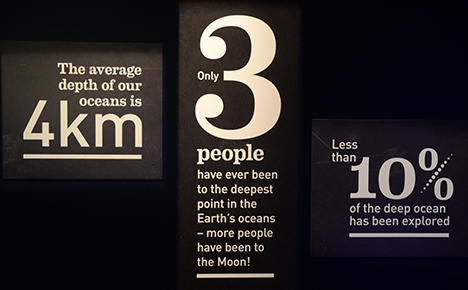## Welcome to H3 Maths

Blog Support for Growing Mathematicians

### The depths that Math goes to…

July7

First, a few basic mathematical facts about our oceans:…which made me think about the equation for water pressure at different depths. Pressure  increases by 1 atm with each 10 m of depth (at sea level the pressure is 1 atm). An “atm” is Atmospheric pressure, which is the force per unit area exerted against a surface by the weight of air above that surface in the Earth’s atmosphere. This increases rapidly. For example, at 2700m below sea level the pressure is nearly 270 times the surface pressure. You can check out these conversions at this useful site:But what does the effect of this increased pressure actually look like? A good illustration, using the concept of scale is shown in this picture of polystyrene heads. The large one is at sea level and the smaller one shows the effect of being at 2700m below sea level! (PS: Polystyrene is a “synthetic polymer made from the monomer styrene, a petrochemical.” – source: Wikipedia)by posted under Uncategorized | Comments Off on The depths that Math goes to…

#### Post Support

The graph on the left (Coronavirus) is for a time period of 30 days, while the one on the right (SARS) is for 8 months! Very poor graphical comparison and hardly relevant, unless it is attempting to downplay the seriousness of the coronavirus?

10 x 9 x 8 + (7 + 6) x 5 x 4 x (3 + 2) x 1 = 2020

NCEA Level 2 Algebra Problem. Using the information given, the shaded area = 9, that is:
y(y-8) = 9 –> y.y – 8y – 9 =0
–> (y-9)(y+1) = 0, therefore y = 9 (can’t have a distance of – 1 for the other solution for y)
Using the top and bottom of the rectangle,
x = (y-8)(y+2) = (9-8)(9+2) = 11
but, the left side = (x-4) = 11-4 = 7, but rhs = y+? = 9+?, which is greater than the value of the opp. side??
[I think that the left had side was a mistake and should have read (x+4)?]